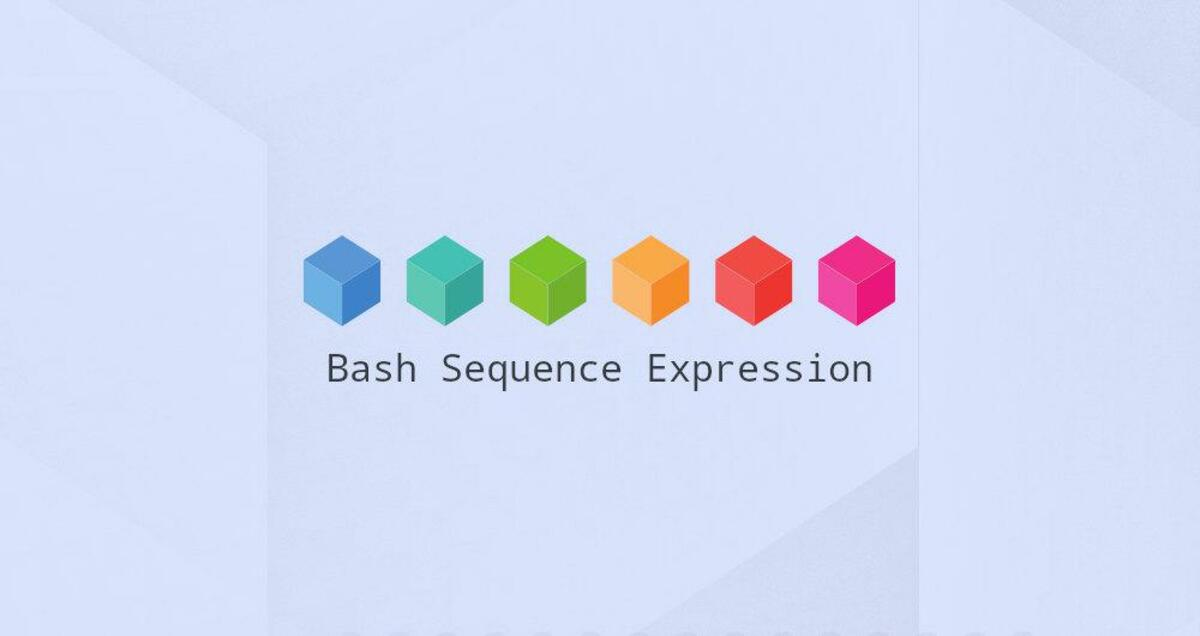The seq command is an abbreviation for sequence and is used to print a sequence of numbers in increasing or decreasing order. In other words, it prints a range of specified values. The numbers can be integers or real numbers with decimal points, or negative numbers. You can also specify the upper or lower limit of the sequence, etc.

In this tutorial, we will explain how to use the seq command and seq common command options, such as printing a sequence of numbers up to the upper limit, printing a specified value between the lower and upper digits, printing a sequence with custom increments, printing a sequence in decreasing or inverted order, printing a sequence with the same number of digits, printing a sequence in a specified format, printing a sequence using a specified separator, and finally we give you a practical application example for your reference.

In its simplest form, you specify an upper limit for the seq and it will print a sequence from 1 to the upper limit.

 ``````1 `````` ``````seq n ``````

Here is an example.

 ``````1 2 3 4 5 `````` ``````➜ myfreax seq 9 1 2 3 4 ``````

You can provide two numbers in ascending order, lower and upper limit values, and seq will print a sequence of numbers from smallest to largest.

 ``````1 `````` ``````seq n1 n2 ``````

Take a look at this example.

 ``````1 2 3 4 5 `````` ``````➜ myfreax seq 3 6 3 4 5 6 ``````

So far, the increment in the sequence has been 1. But you can also customize the increment between the lower and upper limits.

 ``````1 `````` ``````seq n1 inc n2 ``````

The incremental values can be integers or decimals or negative numbers.

 ``````1 2 3 4 5 6 7 8 `````` ``````➜ myfreax seq 3 0.5 6 3.0 3.5 4.0 4.5 5.0 5.5 6.0 ``````

Another trick is to print a sequence in decreasing or inverted order. Therefore, you must specify a negative incremental value.

 ``````1 2 3 4 `````` ``````➜ myfreax seq 6 -1 4 6 5 4 ``````

What happens when you enter 0.7 as the incremental value? In this case, seq will not exceed the upper limit.

 ``````1 2 3 4 5 6 `````` ``````➜ myfreax seq 3 0.7 6 3.0 3.7 4.4 5.1 5.8 ``````

So far, you have not used any of the options of the seq command. Let’s look at and use the seq options.

The seq command’s option `w` is used to keep the printed numbers residing in the same number of digits. You will notice that when not enough values are printed, seq will be prepended with zeros. Examples are as follows.

 ``````1 2 3 4 `````` ``````➜ myfreax seq -w 9 11 09 10 11 ``````

You can use the option `f` to format the output lines in the specified format. Examples are as follows.

 ``````1 2 3 4 `````` ``````➜ myfreax seq -f '##%g##' 3 5 ##3## ##4## ##5## ``````

`%g` for displaying numbers in integer format. `%e` displays numbers in exponential format, `%f` displays numbers in floating point format.

So far, sequences have been printed vertically. This is because by default, the separator is a newline character. You can use the option `s` to specify the separator. An example is shown below.

 ``````1 2 `````` ``````➜ myfreax seq -s ':' 4 1:2:3:4 ``````

The `'` apostrophe in the separator is not required, but it helps avoid shell interpretation. If you use semantic characters for the shell such as `\$`, a wildcard for regular expressions, the shell will interpret the `\$` symbol without the `'` apostrophe.

## Practical application of the seq command

You may be wondering what the practical use of the seq command is. There are many situations where it can be used. One example I can think of is when you are using a for loop in bash. Instead of specifying the sequence manually in the loop condition, you can use the seq command.

 ``````1 2 3 4 5 6 `````` ``````#!/bin/bash for i in \$(seq 4 2 18) do echo "Number \$i" done ``````

When you run the above bash script, it will loop through the specified sequence and print the values.

 ``````1 2 3 4 5 6 7 8 9 `````` ``````➜ myfreax bash seq.sh Number 4 Number 6 Number 8 Number 10 Number 12 Number 14 Number 16 Number 18 ``````

## Conclusion

You already know how to use the seq command and the basic options. seq command will print a series of values between the ones you specify. It can also start with negative numbers, as well as in real-world applications. If you want more details, you can always use its manual page.